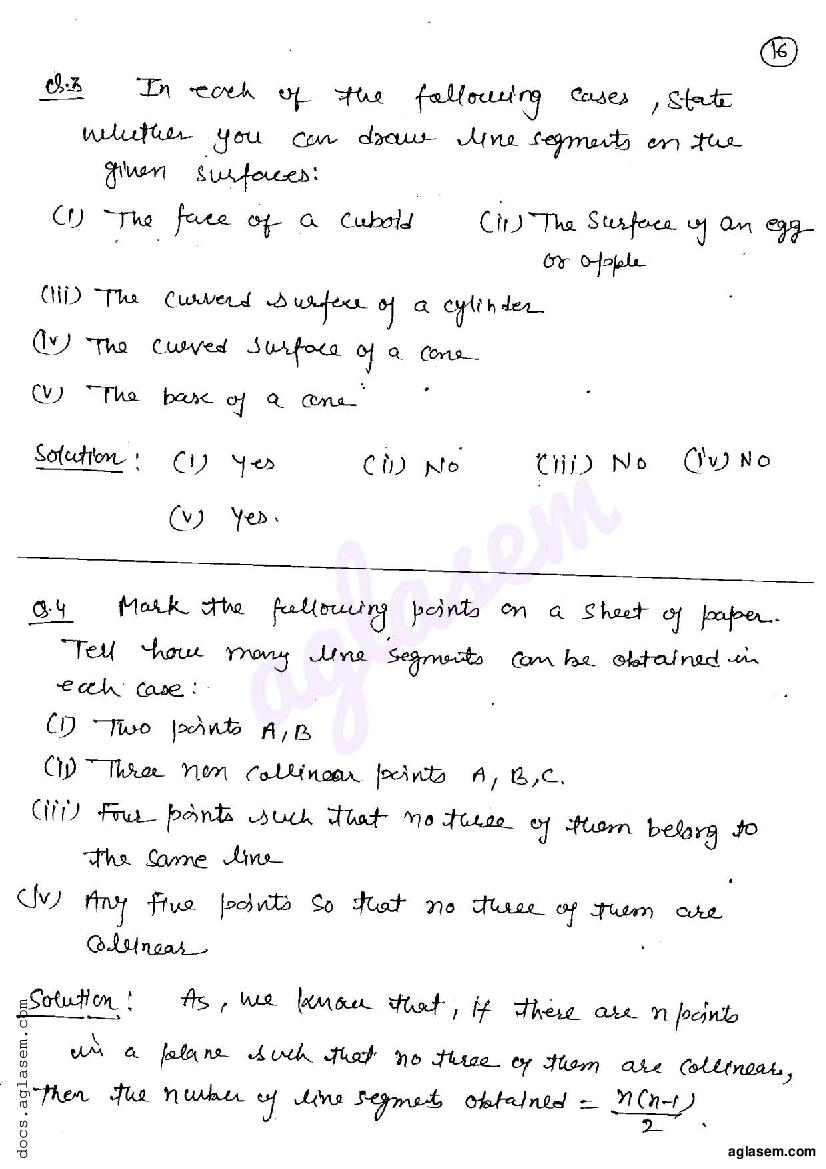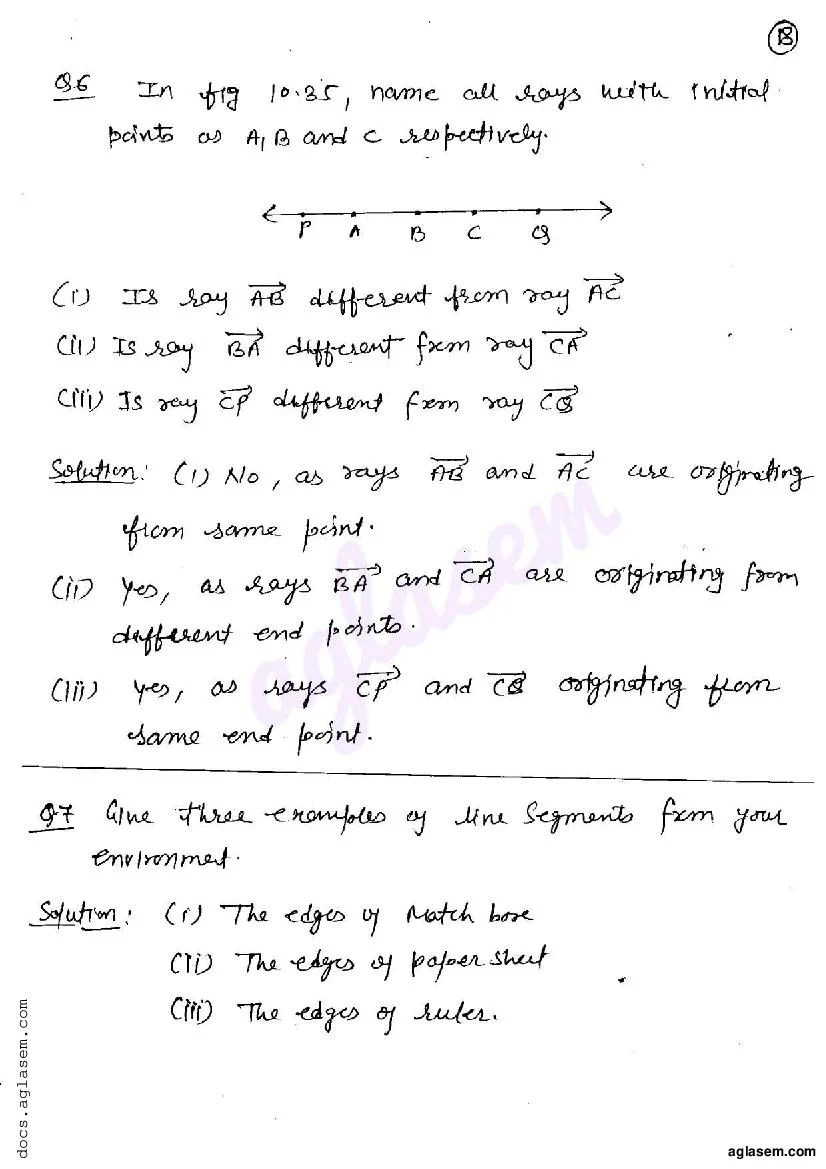# RD Sharma Solutions Class 6 Chapter 10 Basic Geometrical Concepts Exercise 10.2

Here you can get free RD Sharma Solutions for Class 6 Maths Chapter 10 Basic Geometrical Concepts Exercise 10.2. All RD Sharma Book Solutions are given here exercise wise for the chapter Basic Geometrical Concepts. RD Sharma Solutions are helpful in the preparation of several school level, graduate and undergraduate level competitive exams. Practicing questions from RD Sharma Mathematics Solutions for Class 6 Chapter 10 Basic Geometrical Concepts is proven to enhance your math skills.

 Class: Class 6th Chapter: Chapter 10 Exercise: Exercise 10.2 Name: Basic Geometrical Concepts

## RD Sharma Solutions Class 6 Chapter 10 Basic Geometrical Concepts Exercise 10.2

RD Sharma Class 6 Solutions Chapter 10 for Basic Geometrical Concepts Exercise 10.2 are given below.

RD Sharma Solutions Class 6 Maths Chapter 10 Basic Geometrical Concepts Exercise 10.2